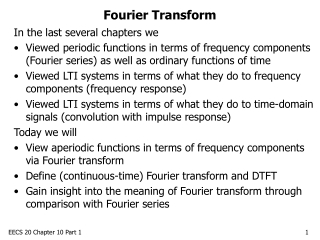DownloadDownload PresentationFourier Transform

# Fourier Transform

Download Presentation## Fourier Transform

- - - - - - - - - - - - - - - - - - - - - - - - - - - E N D - - - - - - - - - - - - - - - - - - - - - - - - - - -
##### Presentation Transcript

1. Fourier Transform In the last several chapters we • Viewed periodic functions in terms of frequency components (Fourier series) as well as ordinary functions of time • Viewed LTI systems in terms of what they do to frequency components (frequency response) • Viewed LTI systems in terms of what they do to time-domain signals (convolution with impulse response) Today we will • View aperiodic functions in terms of frequency components via Fourier transform • Define (continuous-time) Fourier transform and DTFT • Gain insight into the meaning of Fourier transform through comparison with Fourier series EECS 20 Chapter 10 Part 1

2. Review: Fourier Series The Fourier series represents a periodic signal in terms of frequency components: We get the Fourier series coefficients as follows: The complex exponential Fourier coefficients are a sequence of complex numbers representing the frequency component ω0k. EECS 20 Chapter 10 Part 1

3. Discrete Fourier Transform: Like Fourier Series There is another, only slightly different, way to write a discrete-time periodic signal as a sum of complex exponentials of frequency ω0k . We call it the discrete Fourier transform (DFT), but it is very similar to the discrete Fourier series (DFS): We simply move the 1/p term outside the sum. The terms of the DFT are thus similar to the DFS: X’k = p Xk They also give an idea of the relative scaling of the frequency components. EECS 20 Chapter 10 Part 1

4. Discrete Time Fourier Transform Note that for a signal with period p, when determining X’k we just need to sum over 1 period and start the sum at any point: What if the period was really large? Like infinity? Though the DFT is not defined for aperiodic signals, we have a name for the DFT equation extended to an infinite sum: Discrete Time Fourier Transform (DTFT) EECS 20 Chapter 10 Part 1

5. Interpretation of Fourier Transform The discrete-time Fourier transform, like the discrete Fourier series and DFT, gives a measure of the relative weight of each frequency component in the signal x. • But, here we do not compare it to a fundamental frequency ω0 (because there is none). • The fact that periodic signals will only have frequencies that are integer multiples of the fundamental gave us a sequence of possiblefrequencies, and a sequence of weights Xk. • Now we can have any frequency. So we need a function that takes values at all real frequencies to describe the signal. EECS 20 Chapter 10 Part 1

6. Continuous Time Fourier Transform We can extend the formula for continuous-time Fourier series coefficients for a periodic signal to aperiodic signals as well. The continuous-time Fourier series is not defined for aperiodic signals, but we call the formula the (continuous time) Fourier transform. EECS 20 Chapter 10 Part 1

7. Inverse Transforms If we have the full sequence of Fourier coefficients for a periodic signal, we can reconstruct it by multiplying the complex sinusoids of frequency ω0k by the weights Xk and summing: We can perform a similar reconstruction for aperiodic signals: These are called the inverse transforms. EECS 20 Chapter 10 Part 1

8. Example Find the Fourier transform of a pulse centered at zero: 1 -1 1 EECS 20 Chapter 10 Part 1

9. Sinc Function EECS 20 Chapter 10 Part 1

10. Fourier Transform of Impulse Functions Find the Fourier transform of the Dirac delta function: Find the DTFT of the Kronecker delta function: The delta functions contain all frequencies at equal amplitudes. Roughly speaking, that’s why the system response to an impulse input is important: it tests the system at all frequencies. EECS 20 Chapter 10 Part 1

11. The Heisenberg Uncertainty Principle The delta functions are “localized” in time; they are nonzero at just one point and zero everywhere else. But the frequency “spread” of the delta functions is not localized. We showed that X(ω) is always 1; it never dies out. For sinusoids, the opposite is true. They never die out in time, but the frequency spread is just one point. The pulse function was somewhat localized in time, and somewhat localized in frequency (the sinc function dies out asymptotically). This is the Heisenberg uncertainty principle: the product of the time “spread” and frequency “spread” of a function can never be less than a defined minimum nonzero value. EECS 20 Chapter 10 Part 1

12. Time, Frequency, and Time-Frequency We know we can represent functions in terms of frequency components (sinusoids). These basis functions are nonzero at single points in the frequency domain, but never die out in the time domain. We can also represent functions in the time domain. Using the sifting property, we can represent any function in terms of deltas. For example, imagine every discrete time function as a train of appropriately scaled Kronecker deltas. These basis functions are single points in time, never dying out in frequency. We can also represent functions in terms of other basis functions, somewhat localized in time and frequency, like the pulse and sinc. These functions are referred to as wavelets, and they form time-frequency representation of a signal. EECS 20 Chapter 10 Part 1